SPSS TUTORIALS BASICS DATA ANALYSIS REGRESSION ANOVA T-TESTS

# SPSS Time Variables Tutorial

Having a solid understanding of what SPSS time variables are, you may find calculations on them surprisingly easy. This tutorial will demonstrate SPSS' main time functions. However, we'll also show that we often don't even need them for getting things done.
Throughout this tutorial, keep in mind that SPSS time variables contain time spans in numbers of seconds that may or may not express clock times. Second, time variables are numeric variables so all numeric functions can be applied to them.
We encourage you try the time calculations we'll demonstrate yourself. You can do so by downloading and opening clock_card.sav.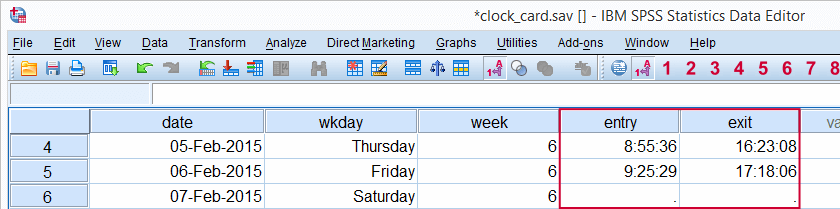## SPSS Main Time Functions

Most of SPSS' date functions are intended for time variables as well. After outlining them in the table below, we'll take a closer look at them in the remainder of this tutorial.

FunctionUseExampleReturns
DATEDIFFCompute difference between two times in given time unitDATEDIFF(time1,time2,'minutes')Standard numeric value
DATESUMAdd number of given time units to time variableDATESUM(time,8,'hours')Time value
XDATEExtract time component from time variableXDATE.HOURS(time)Standard numeric value
TIME.HMSCreate time value from hours, minutes, secondsTIME.HMS(20,15,30)Time value

## SPSS DATEDIFF Function

Our data contain the entry and exit times of an employee as registered with a clock card. We first want to know how much time he spent in the office per day. The syntax below shows how to do so with and without using DATEDIFF. The screenshots show the results of both options.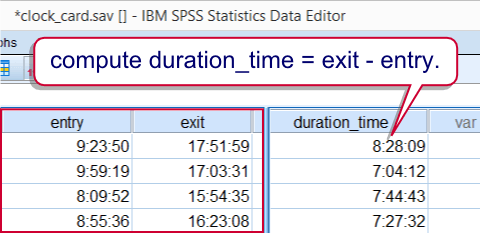## SPSS DATEDIFF Syntax Example

*1. Compute duration in seconds.

compute duration_time = exit - entry.
exe.

*2. Display duration in seconds as time.

formats duration_time(time8).

*2. Compute duration in minutes with DATEDIFF.

compute duration_minutes = datediff(exit,entry,'minutes').
exe.

*4. Hide decimals.

formats duration_minutes(f3).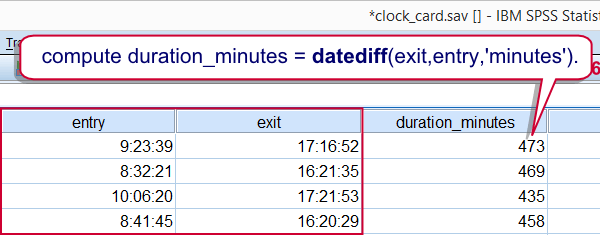## SPSS DATESUM Function

Employees are supposed to spend 8 hours per day in the office. That is, their entry times should be their exit times minus 8 hours. Such time subtractions (or additions) are easily accomplished by using DATESUM. However, realizing that hours consist of 3600 seconds, we may obtain the same result with an ordinary addition as shown in the second example.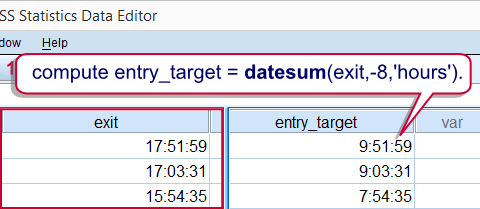## SPSS DATESUM Syntax Example

*1. Compute entry_target (8 hours before leaving) in seconds.

compute entry_target = datesum(exit,-8,'hours').
exe.

*2. Display entry_target as time.

formats entry_target(time8).

*3. Alternative to datesum for exit_target (8 hours after entry).

compute exit_target = entry + 3600 * 8.
exe.

*4. Display exit_target as time.

formats exit_target(time8).

## SPSS XDATE Function

Employees are supposed to be in before 10 AM. One way to flag late entries is to extract the hours from the entry times with XDATE. XDATE needs to be suffixed with the time unit we wish to extract as in XDATE.HOURS. Finally, we'll RECODE the hours into our flag variable.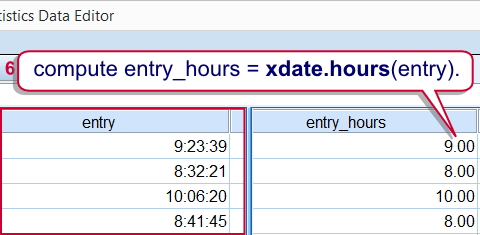## SPSS XDATE Syntax Example

*1. Extract hours from entry.

compute entry_hours = xdate.hours(entry).
exe.

*2. Flag cases where entry_hours >= 10 (late entry).

recode entry_hours(lo thru 9 = 0)(10 thru hi = 1) into late_entry.
exe.

## SPSS TIME.HMS Function

SPSS time variables hold numbers of seconds. TIME.HMS converts a number of hours, minutes and seconds into seconds and is thus creates SPSS time values from normal time components.
The minutes and seconds are optional; if omitted, they'll default to zero. That is, TIME.HMS(10) is a shorthand for TIME.HMS(10,0,0) and returns 36,000 (seconds). We can show this value as 10:00:00 by setting its format to TIME8.
The syntax below uses TIME.HMS as an alternative way to flag late entries.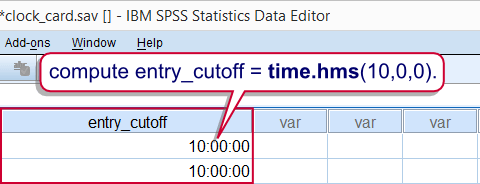## SPSS TIME.HMS Syntax Example

*1. Compute entry_cutoff as 10 AM.

compute entry_cutoff = time.hms(10,0,0).
exe.

*2. Display entry_cutoff as time.

formats entry_cutoff(time8).

*3. Delete late_entry before recalculating it.

delete variables late_entry.

*4. Recalculate late_entry.

if entry < entry_cutoff late_entry = 0.
if entry >= entry_cutoff late_entry = 1.
exe.

## SPSS Time Comparisons

SPSS time comparisons are utterly simple when we realize that SPSS time values are just numbers of seconds that are shown as hours, minutes and seconds. For comparing an SPSS time value to a normal time value (hours, minutes and seconds), simply convert the latter into seconds. TIME.HMS does just that. Next, simply use SPSS' standard operators such as >, <= and others.
For example, employees are not supposed to leave before 4 PM. The syntax below shows a super shorthand for flagging early exits.The unusual COMPUTE command is explained in Compute A = B = C.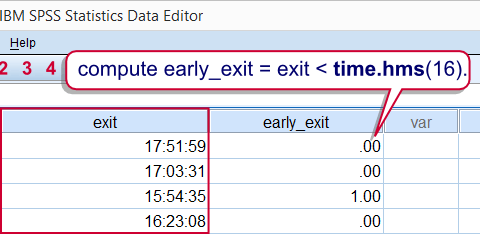## SPSS Time Comparison Syntax Example 1

*Super shorthand for flagging early exits (before 4 PM).

compute early_exit = exit < time.hms(16).
exe.

## SPSS Time Comparison Example 2

Because TIME.HMS is a function, it can be substituted in other functions, particularly RANGE. The following example shows how to use it for flagging entries during rush hours (from 8 until 9 AM).

*Flag entry times between 8 and 9 with RANGE.

compute rush_hour_entry = range(entry,time.hms(8),time.hms(9)).
exe.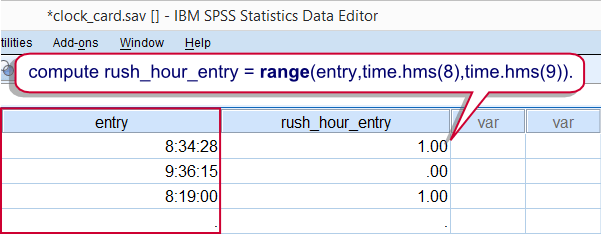## SPSS Time Variables in AGGREGATE

This final example again reemphasizes that SPSS time variables are numeric variables, holding seconds, on which normal numeric functions can be used.
For example, employees are supposed to work 40 hours per week. To what extent do our data meet that criterion? We already calculated duration_time, which is a time variable holding seconds. We can simply sum it per week by using AGGREGATE. This results in seconds per week which we'll show as normal times by setting their format to TIME8.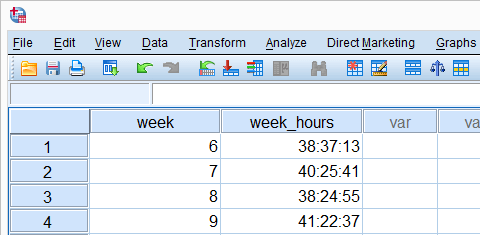*1. Compute seconds in the office per week.

aggregate outfile *
/break week
/week_hours = sum(duration_time).

*2. Show seconds as hours, minutes, seconds.

formats week_hours(time8).

# Tell us what you think!

*Required field. Your comment will show up after approval from a moderator.

# THIS TUTORIAL HAS 12 COMMENTS:

•### By Maiko Nicholas on October 13th, 2015

Easy to follow procedures...

•### By Ruben Geert van den Berg on October 13th, 2015

Thanks! Yes, I agree. Once you realize how SPSS stores date, time and datetime variables, all sorts of time calculations become as easy as basic additions and subtractions.

•### By Anna on December 21st, 2015

Thanks for a nice tutorial!
However, I had a question about when you have timepoints at different dates. I want to calculate the time between an hour:minute timepoint day 1, and an hour:minute timepoint day 2. For example Day 1: 20:30 to Day 2: 08:00, which should be 11:30. Do you know of a way to do this in SPSS?

•### By Ruben Geert van den Berg on December 22nd, 2015

Sure! Combine the date and time variables into datetime variables. If you think about it for a minute, you may conclude that time variables without dates are somewhat odd in the first place. Datetime variables are the real deal!

The relation between SPSS date, time and datetime variables makes perfect sense. As soon as you understand it, working with datetime functions will be perfectly straightforward as well.

Hope that helps!

•excelent